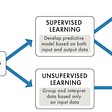The benefit of Numerical Gradient Check(NGC) is designed to manually verify that the deep neural network(DNN) is doing the right partial derivatives in the back-pass(BP: Backpropagation in other papers, we call this back-pass in this article).

## Understanding DNN’s BP

The whole process of DNN is actually to get that the difference between the predicted outcome of the model and the fact after one training round is an unpredictable minimum, the process could be a mini-batch or an EPOCH.

The “minimum” refers to the maximum likelihood of an exponential family of functions called the cost function (CF: some framework like Tensorflow calls loss).

So, the CF is a function of the model, which means the function of the parameters of the model.

That is, each parameter affects the difference between the predicted and true values. Mathematically it is the partial derivative of each parameter with respect to CF, right?

## Illustrations

Since we know that the parameter is a function of loss or cost, the extremum is the tangent line (middle school knowledge, right?).

So the tangent line at loss(w) is the derivative of CF (loss) respected by w.

## Goal of NGC

How to verify that our DNN is using the proper derivative operations at w correctly, then we need to compare the results of the computer run (DNN loop) with the manually calculated results, and the difference between them should be very small.

The idea is fairly intuitive. We take a value w-e that is smaller than the w slice, calculate its function loss(w-e) for CF, and then take a value w+e that is larger than the w slice, calculate its function loss(w+e) for CF so that the slope of the two points should be very close to the derivative of w itself for the cost (the derivative of CF).

(e: 1e-4 which is an experienced Value)

## Design a simple DNN to demo (just a linear regression)

1. Input with five features x1~x5.
2. Implicts that there are w1~w5 parameters in a neuron in the next layer.
3. We give only one neuron in the next layer, and this next layer is the final output layer.
4. Also, give w0 for bias and later give an x0 which is always 1 to support the w0. (This is a very trick and my favorite approach learned from Prof. Ng).
5. Give some initializations of model parameters including bias (INIT).
6. Defining gradient descent (GD), giving the formula directly is not the subject of this paper.
∂ CF / ∂ W = X_T * (Y_hat-Y)
7. Run one-step GD with INIT, save all decent values.
8. Pitch each value of INIT, calucate slop of each by
[loss(w+e)-loss(w-2)] / (2*e)
9. The deviation between step 7. and step 8. must be very small.

# I’m not good at writing too much detail, here’s a copy of the code, and the notes in it say it all.

https://gist.github.com/XinyueZ/eae3e9b813f6b5d55f0d85f9c386bb96

--

--

--

## More from TeeTracker

Advocate, Enthusiast: AI, machine learning, deep learning

Love podcasts or audiobooks? Learn on the go with our new app.

## End-to-end Object Detection Using EfficientDet on Raspberry Pi 3 (Part 2)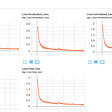## How to create a machine learning model from scratch with CreateML## Catalyst dev blog - 20.07 release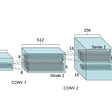## Learning Logistic Regression## Applying Machine Learning to Optimization Projects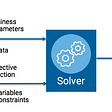## Learning with Small Data: Part 2## Legal Certainty and the Possibility of Computer Decision Making in the Courtroom## TeeTracker

Advocate, Enthusiast: AI, machine learning, deep learning

## Hello, Neural Networks!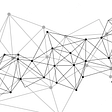## In-Depth Review of Convolutional Neural Networks (CNN’s)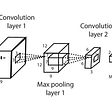## Confusion Matrix without Confused## Why initialize weights randomly(numpy.random.randn()) in Hidden Layered Neural Networks ?